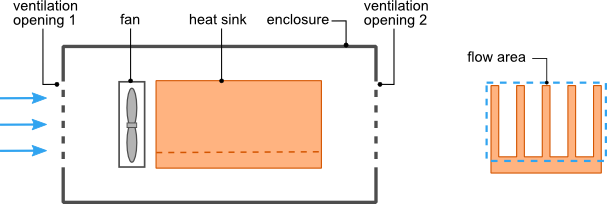31 Aug

There are many situations in which the heat sink and the device(s) it is cooling are located in an enclosure with ventilation openings. The ventilation openings will allow the inlet of air at the outside ambient temperature and the exit of heated air. When the heat sink is being cooled by forced convection using a fan or blower the size and configuration of the ventilation openings can negatively impact the ability of fan/heat sink to cool the power generating devices when compared to the same fan/heat sink combination located in an open environment outside of the enclosure. This is because the ventilation openings which are typically perforated plates, louvered openings or mesh screens restrict the flow of air. Understanding what impact this restriction has on the volumetric flow rate of the air through the heat sink will allow you to select a heat sink and fan combination to meet the thermal requirements of the device being cooled.

Shown in figure 1 is an enclosure with ventilation openings at both ends. There is a heat sink inside the enclosure with a fan mounted on or very close to the heat sink such that it can be assumed that all of the air flow from the fan will pass through the heat sink.Figure1.  Heat sink and fan in a ventilated enclosure

As the air flows through the ventilation openings and the heat sink there is a drop in pressure across each component. In order analyze the flow through the enclosure we will apply rules which are analogous to the analysis of an electric circuit. Figure 2 shows the network representation of the flow through the enclosure. The pressure source Pfan represents the pressure increase created by the fan, Rvent1, Rvent2 and Rhs are the flow resistances of the ventilation openings and heat sink respectively.  The fan is analogous to a voltage source and flow resistances analogous to electrical resistances in an electric circuit.

The sum of the pressure differences around the flow network loop must be equal to zero.$Latex formula$

For a detailed explanation of how the pressure drop across a heat sink based on rate flow rate is calculated refer to the post Heat Sink Design Optimization for Forced Convection.

The variation of pressure across the fan as a function of the volumetric flow rate can be obtained from the fan flow curve provided by the fan manufacturer. The flow curve will be provided as a graph or data points of pressure difference across the fan versus volumetric flow rate.

To calculate the pressure drop across the ventilation openings equations obtained from Idelchik  are used. The equation show below for$Latex formula$ calculates the pressure drop for a thin perforated plate as shown in figure 3. The equations for the pressure drop across the heat sink are dependent on the spacing between the fins, height of the fins and the volume flow rate through the heat sink. These equations will not be shown here. Consult Culham et al.   for a detailed explanation of the equations and methodology used to calculate the pressure drop across a plate fin rectangular heat sink .$Latex formula$ is the volumetric flow rate through the ventilation openings$Latex formula$ is the total area of the ventilation$Latex formula$ is the total open area formed by the ventilation holes$Latex formula$ is the area of a single ventilation opening$Latex formula$ is the perimeter of a single ventilation opening$Latex formula$

Note:$Latex formula$ can be calculated for openings of arbitrary shape. For circular openings$Latex formula$ the diameter of the circle.

The pressure drop across the ventilation openings is given by$Latex formula$

where:$Latex formula$ is the density of air$Latex formula$ is the velocity of the air through the ventilation openings given by:$Latex formula$

for Re>10^5$Latex formula$

for 30<Re<10^5$Latex formula$

for Re<10$Latex formula$$Latex formula$ is the Reynolds number of the opening calculated using the following equation:$Latex formula$

where:$Latex formula$ is the kinematic viscosity of air

The values for$Latex formula$ and$Latex formula$ are provided in tabulated form in . In order to conveniently calculate these two variables the tabulated values were fit to analytical functions using regression analysis. The fitted functions are shown below.$Latex formula$

We wish to calculate the volume flow rate Q so that can be used to calculate the thermal resistance of the heat sink and the temperature of the device being cooled. This is done by substituting the pressure drop across the ventilation openings, the appropriate equation of the pressure drop across the heat sink and a linearized form of the fan curve data into equation for the sum pressure differences around the flow network loop and solving for Q. This can done with MSExcel, MathCad or using some other numerical solver.

In part 2 of this article an explanation of how a modified fan curve can be used in the HeatSinkCalculator to analyze a heat sink within a ventilated enclosure will be presented.

References:
 I.E. Idelchik, “Handbook of Hydraulic Resistance, 2nd edition”, Hemisphere Publishing Corporation, New York NY

 J.R. Culham, Y.S. Muzychka “Optimization of Plate Fin Heat Sinks Using Entropy Generation Minimization”, in: IEEE Transactions on Components and Packaging Technology, Vol 24, No.2, pp. 34-41, 2001